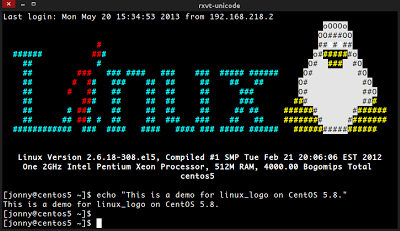### [完全用 GNU/Linux 工作] 09. Hacking Bash▲ 原生的 Bash (on CentOS)。

### 1. 補完自動補齊功能

1.1. 安裝
[ jonny@linux ~ ]
# Debian, Ubuntu
\$ sudo aptitude install bash-completion Enter

# CentOS with epel repo.
\$ sudo yum install bash-completion Enter

1.2. 使用
[ jonny@linux ~ ]
# Debian, Ubuntu
\$ sudo aptitude <Tab> <Tab> <Tab> ... Enter

# CentOS, RHEL, Fedora
\$ sudo yum <Tab> <Tab> <Tab> ... Enter

### 2. 凍仁的環境設定

2.1. 下載凍仁的環境設定。
[ jonny@wheezy ~ ]
\$ git clone https://github.com/chusiang/tuxENV.git Enter

2.2. 切換目錄。
[ jonny@wheezy ~ ]
\$ cd tuxENV/ Enter

2.3. 備份原設定。
[ jonny@wheezy ~ ]
\$ make backup Enter

2.4. 安裝。
[ jonny@wheezy ~ ]
\$ make install Enter

2.5. 將語系指定成英文語系 (en_US.UTF-8) ：因 tty (Ctrl + Alt + F1) 底下預設不支援中文，故新增此設定，適合進階使用者。
# - language
tty=`tty`
t=`echo \$tty|awk -F "/" '{print \$3}'`
if [ "\$(tty)" = "/dev/tty1" ] || [ \$t = pts ]; then
export LANGUAGE="en_US.UTF-8"
export LANG="en_US.UTF-8"
export LC_ALL="en_US.UTF-8"
fi

2.6. ls 顏色設定：部份的 GNU/Linux 使用 ls 時的顏色不易閱讀，故手動更改。
export LS_COLORS='rs=0:di=01;34:ln=01;36:mh=00:pi=40;33:so=01;35:do=01;35:bd=40;33;01:cd=40;33;01:or=40;31;01:su=37;41:sg=30;43:ca=30;41:tw=30;42:ow=34;42:st=37;44:ex=01;32:*.tar=01;31:*.tgz=01;31:*.arj=01;31:*.taz=01;31:*.lzh=01;31:*.lzma=01;31:*.tlz=01;31:*.txz=01;31:*.zip=01;31:*.z=01;31:*.Z=01;31:*.dz=01;31:*.gz=01;31:*.lz=01;31:*.xz=01;31:*.bz2=01;31:*.bz=01;31:*.tbz=01;31:*.tbz2=01;31:*.tz=01;31:*.deb=01;31:*.rpm=01;31:*.jar=01;31:*.war=01;31:*.ear=01;31:*.sar=01;31:*.rar=01;31:*.ace=01;31:*.zoo=01;31:*.cpio=01;31:*.7z=01;31:*.rz=01;31:*.jpg=01;35:*.jpeg=01;35:*.gif=01;35:*.bmp=01;35:*.pbm=01;35:*.pgm=01;35:*.ppm=01;35:*.tga=01;35:*.xbm=01;35:*.xpm=01;35:*.tif=01;35:*.tiff=01;35:*.png=01;35:*.svg=01;35:*.svgz=01;35:*.mng=01;35:*.pcx=01;35:*.mov=01;35:*.mpg=01;35:*.mpeg=01;35:*.m2v=01;35:*.mkv=01;35:*.webm=01;35:*.ogm=01;35:*.mp4=01;35:*.m4v=01;35:*.mp4v=01;35:*.vob=01;35:*.qt=01;35:*.nuv=01;35:*.wmv=01;35:*.asf=01;35:*.rm=01;35:*.rmvb=01;35:*.flc=01;35:*.avi=01;35:*.fli=01;35:*.flv=01;35:*.gl=01;35:*.dl=01;35:*.xcf=01;35:*.xwd=01;35:*.yuv=01;35:*.cgm=01;35:*.emf=01;35:*.axv=01;35:*.anx=01;35:*.ogv=01;35:*.ogx=01;35:*.aac=00;36:*.au=00;36:*.flac=00;36:*.mid=00;36:*.midi=00;36:*.mka=00;36:*.mp3=00;36:*.mpc=00;36:*.ogg=00;36:*.ra=00;36:*.wav=00;36:*.axa=00;36:*.oga=00;36:*.spx=00;36:*.xspf=00;36:'

2.7. 別名 (Alias)。
alias la='ls -A'
alias ll='ls -l'

2.8. 自訂 PS1 顏色：除了色彩較為豐富，也可用來辨識機器，以避免關錯電腦。
# == Colors ==
red='\[\033[0;31m\]'
RED='\[\033[1;31m\]'
green='\[\033[0;32m\]'
GREEN='\[\033[1;32m\]'
yellow='\[\033[0;33m\]'
YELLOW='\[\033[1;33m\]'
blue='\[\033[0;34m\]'
BLUE='\[\033[1;34m\]'
purple='\[\033[0;35m\]'
PURPLE='\[\033[1;35m\]'
cyan='\[\033[0;36m\]'
CYAN='\[\033[1;36m\]'
white='\[\033[0;37m\]'
WHITE='\[\033[1;37m\]'
NC='\[\033[0m\]' # No Color

PS1="\${white}[ \${YELLOW}\u\${RED}@\h \${PURPLE}\w \${white}] - \A \n\${WHITE}\\$ \${NC}"▲ 自訂 PS1 後的 Bash (Debian)。。

2.9. 使用 Vi 模式控制 Bash，預設為 Emacs 模式。
[ jonny@wheezy ~ ]
set -o vi Enter

### 3. 快捷鍵

3.1. 快速搜尋使用過的指令。
ESC + /
Ctrl + r

3.2. 往前刪除一字元，同 Backspace 鍵。
Ctrl + h

3.3. 送出確認／回傳，同 Enter 鍵。
Ctrl + j

3.4. 清除游標以前的一個單字。
Ctrl + w

3.5. 清除游標以前所有的字完。
Ctrl + u

### 4. 指令簡介

#### 4.1. 常用指令

[ jonny@wheezy ~ ]
```\$ history | awk '{print \$2}' | sort | uniq -c | sort -nr | nl | less Enter
1     2971 ls
2     2236 cd
3     1843 git
4     1180 sudo
5      484 cat
6      354 mv
7      354 gpg
8      322 vi
9      304 ssh
10      255 cp```

4.1.1. ls: 列出檔案及目錄。
[ jonny@wheezy ~ ]
\$ ls Enter
bin public_html tmp vcs

4.1.2. cd: 切換目錄。
[ jonny@wheezy ~ ]
\$ cd /tmp Enter
[ jonny@wheezy /tmp ]
\$

4.1.3. git: 可以讓檔案進行儲存、分支的分散式版本控制系統，好比玩單機遊戲時我們會先儲存以得到最美好的結局。

4.1.4. sudo: 暫時取得管理者權限。建議平時別用超級管理者登錄 (root)，待有需要時再用 sudo，可有效降低人為失誤的風險。
[ jonny@wheezy ~ ]
\$ sudo /etc/init.d/nginx restart Enter
Restarting nginx: nginx.
[ jonny@wheezy ~ ]
\$

4.1.5. cat: 查看檔案內容。
[ jonny@wheezy ~ ]
\$ cat /etc/issue Enter
Raspbian GNU/Linux 7 \n \l

4.1.6. mv: 搬移檔案及目錄。
[ jonny@wheezy ~ ]
\$ ls Enter
1234567
[ jonny@wheezy ~ ]
\$ mv 1234567 7654321; ls Enter
7654321

4.1.7. gpg: 加密、數位簽章及產生非對稱式金鑰的軟體，本次篇幅不會提到。

4.1.8. vi: 使用 H, J, K, L 取代左、下、上、上移動的純文字編輯器。

4.1.9. ssh: 遠端連線。
[ jonny@wheezy ~ ]
\$ ssh jonny@192.168.11.6 Enter
Linux raspberrypi 3.6.11+ #538 PREEMPT Fri Aug 30 20:42:08 BST 2013 armv6l

The programs included with the Debian GNU/Linux system are free software;
the exact distribution terms for each program are described in the

Debian GNU/Linux comes with ABSOLUTELY NO WARRANTY, to the extent
permitted by applicable law.
Last login: Thu Sep 26 23:19:56 2013 from 192.168.11.9
[ jonny@wheezy ~ ]
\$

4.1.10. cp: 複製檔案。
[ jonny@wheezy ~ ]
\$ ls Enter
tux.txt
[ jonny@wheezy ~ ]
\$ cp tux.txt helloTux.txt Enter
[ jonny@wheezy ~ ]
\$ ls Enter
helloTux.txt tux.txt

#### 4.2. 進階指令

4.2.1. 將使用 markdown 語法 (07.ufw.md) 的文件轉換成 HTML 格式 (markdown_py)，並導向 (>) 指定的檔案 (07.ufw.html)。
[ jonny@wheezy ~ ]
\$ markdown_py 07.ufw.md > 07.ufw.html Enter

4.2.2. 檢視 (cat) 檔案，並將結果丟給管線 (pipe) 後的程式處理 (xlip: 複製內容至剪貼簿)。
[ jonny@wheezy ~ ]
\$ cat 07.ufw.html | xclip Enter

4.2.3. 透過 grep 過濾出已安裝的套件。
[ jonny@wheezy ~ ]
\$ sudo aptitude search vim | grep ^i Enter
```i   vim                  - Vi IMproved - enhanced vi editor
i   vim-common           - Vi IMproved - Common files
i   vim-gnome            - Vi IMproved - enhanced vi editor - with GN
i A vim-gui-common       - Vi IMproved - Common GUI files
i A vim-runtime          - Vi IMproved - Runtime files
i   vim-syntax-gtk       - Syntax files to highlight GTK+ keywords in
i   vim-tiny             - Vi IMproved - enhanced vi editor - compact```

```相關連結： ★bash shell 入門 第三版 | O'Reilly ★凍仁的筆記: bash ★凍仁的筆記: Shell Scripts ```